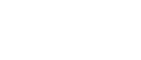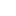#• PRODUCTS• SOLUTIONS• TUTORIALS• SUPPORTTaiwan | English• Japan
• Global
• United States
• Europe
• China
• Korea
• Taiwan
• India

#• PRODUCTS
• SOLUTIONS
• TUTORIALS
• SUPPORT

# Precise position measurement

Explanation of AKM's magnetic sensing technology

• Precise position detection with a Hall element
• Recommended products for application

Magnetic Sensing

Detection of very small displacement or close position is required as a result of recent developments in miniaturization and advances in the functionality of equipment.

We have developed the industry’s smallest micro Hall element for precise position detection using a Hall sensor. Here, we introduce an example of precise position detection achieved through use of a Hall element.

## Precise position detection with a Hall element

Example:

Using a Hall element with analog output makes it possible to detect the position of an object by resolving power in the order of a few micrometers for a stroke of 1 mm (moving distance).

Detection by resolving power in the order of a few micrometers is made possible by arranging two sensors and computing both outputs for a stroke of a few millimeters (moving distance).

In this case, the thermal characteristics of the two sensors are canceled, and linear output is obtained. If a product with two built−in sensors (one package) is used, mounting error is not be an issue, and handling is easy.

## Advantages of performing precise position detection using a Hall element

• Extended durability due to non−contact detection.
• Because magnetism is detected, the device is more resistant to tiny particles of dirt, dust, or oil, compared to optical devices.
• Miniaturization is possible.

## Principle

This principle enables a Hall element to achieve output proportional to the magnetic flux density. By designing a layout where the magnetic flux density changes proportionally to the displacement of the measured object at the position of the Hall element, a Hall effect output proportional to the displacement can be obtained.

## Example of composition

Here are two typical examples and one composition peculiar to the Hall element in which flux density distribution proportional to the displacement is obtained.

### Composition 1-1

This is the simplest composition, with one magnet and one Hall element. Because detection is performed using the range near the zero crossing (zero magnetic field), the output is comparatively small.

< Feasible stroke and accuracy >

● Stroke: 1mm

●Linearity: ±1μm

### Composition 1-2

This consists of two magnets (from the Hall element side, two pole magnetized) and one Hall element. Because the direction of magnetization is vertical to the detection surface of the Hall element, the magnetic flux density is comparatively high, and a relatively high output can be obtained. If the size of the magnet is small, the range in which linear output can be obtained is limited because the detection range of the magnetic flux is small.

< Feasible stroke and accuracy >

● Stroke: 1mm

● Linearity: ±1μm

### Composition 2

This example comprises one magnet and two Hall elements.

● Utilizing the fact that the outputs of two Hall elements make a mirror image (overlap when folded), it is composed so that the difference in outputs of two Hall elements becomes linear with the displacement.

● The output from the Hall element is large, and relatively large displacement can be covered.

Furthermore, the thermal characteristics of the output voltage are canceled by dividing the difference in the outputs by the sum of the outputs.

< Feasible stroke and accuracy >

● Stroke: 1 to 5mm

● Linearity: 1 to 5um

### Composition 3

This example comprises one magnet and two Hall elements.

● Same as Composition-3, the difference in outputs of two Hall elements becomes linear with the displacement.

● Skewed magnet makes the difference in outputs of two Hall elements linear in wide range.

● Large displacement can be covered by big outputs of Hall elements.

Furthermore, the thermal characteristics of output voltage are cancelled by dividing the difference in the outputs by the sum of the outputs.

< Feasible stroke and accuracy >

● Stroke: 5 to 10mm

● Linearity: 30 to 50um